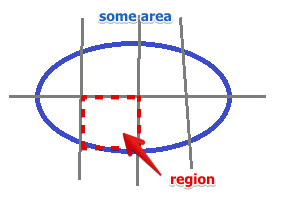# ML Wiki

## Parliamentary Allocation

Suppose we run an election. We want to allocate seats in the parliament proportionally to the number of votes each party received.

Problem:

• $n$ - number of voters that participate in the elections
• $N$ - number of classes (parties) that are being elected
• $P_1, ..., P_k$ - are partitions of the population, \sum_P = N
• size of $P_i$ is $p_i$
• goal: to select $S$ representatives, $S$ - the total number of seats that are allocated

A quota $q_i$ of party $i$ is the number of sets the party receives after election:

• $q_i = S \cdot \cfrac{P_i}{n}$
• but it must be an integer! Cannot divide one seat between two parties

This is important not only for seats allocation, but for allocating in general.

For example,

• suppose we have some area that suffers from fire
• we have only 10 medical units
• how to allocate them between the regions in that area?
•• can allocate as follows: the number of voters $p_i$ - density of a region, $n$ - all people of the area
• $S$ is the number of medical units
• $q_i$ the number of medical units sent to region $i$
• $q_i = S \cdot \cfrac{P_i}{n}$ - again: it has to be an integer!